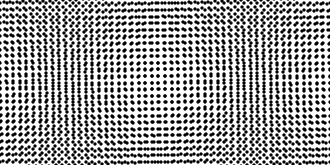Search IntMath
Close

450+ Math Lessons written by Math Professors and Teachers

5 Million+ Students Helped Each Year

1200+ Articles Written by Math Educators and Enthusiasts

Simplifying and Teaching Math for Over 23 Years

# 2D Shapes in Geometry## What are 2D Shapes in Geometry?

2D shapes in geometry are two-dimensional shapes. They have length and width, but no depth. 2D shapes can be found in many different forms and can be used to construct objects in the real world, as well as in mathematics. 2D shapes are also known as polygons, and they can be regular or irregular.

2D shapes can be categorized into different types, including circles, squares, rectangles, triangles, trapezoids, parallelograms, rhombuses, and kites. Each type of 2D shape has its own set of properties and characteristics, which can be used to identify them.

## Circles

Circles are round, two-dimensional shapes that have a radius, or distance from the center to the edge. Circles are important in geometry since they are used to measure angles and distances. The area of a circle is calculated using the formula A = pr2, where A is the area and r is the radius of the circle.

Circles can also be used to construct objects in the real world. For example, a wheel is a circular object that is used to move things from one place to another.

## Squares

Squares are four-sided polygons that have all four sides equal in length and all four angles equal in measure. The area of a square is calculated using the formula A = s2, where A is the area and s is the length of one side of the square.

Squares are used to construct objects in the real world. For example, a cube is a three-dimensional object that is made up of six equal squares.

## Triangles

Triangles are three-sided polygons that have three sides of varying lengths and three angles of varying measure. The area of a triangle is calculated using the formula A = 1/2 bh, where A is the area, b is the base of the triangle, and h is the height of the triangle.

Triangles are also used to construct objects in the real world. For example, the roof of a house is typically made up of multiple triangles.

## Practice Problems

1. Find the area of a circle with a radius of 5 cm.

Answer: A = pr2 = p(5 cm)2 = 78.5 cm2

2. Find the area of a square with a side length of 6 cm.

Answer: A = s2 = (6 cm)2 = 36 cm2

3. Find the area of a triangle with a base of 8 cm and a height of 10 cm.

Answer: A = 1/2 bh = 1/2 (8 cm)(10 cm) = 40 cm2

4. Find the circumference of a circle with a radius of 7 cm.

Answer: C = 2pr = 2p(7 cm) = 43.98 cm

5. Find the perimeter of a square with a side length of 5 cm.

Answer: P = 4s = 4(5 cm) = 20 cm

6. Find the perimeter of a triangle with sides of length 8 cm, 10 cm, and 12 cm.

Answer: P = 8 cm + 10 cm + 12 cm = 30 cm

## Summary

In this article, we discussed 2D shapes in geometry. These shapes can be categorized into different types, including circles, squares, rectangles, triangles, trapezoids, parallelograms, rhombuses, and kites. Each type of 2D shape has its own set of properties and characteristics, which can be used to identify them. We also practiced some problems related to finding the area and perimeter of different 2D shapes.

## FAQ

### What is a 2D shape in geometry?

A 2D shape in geometry is a shape that has two dimensions, such as length and width. It is flat and can be measured and drawn on a plane.

### What is the definition of 2D figures?

A 2D figure is a shape that has two dimensions, such as length and width. It is flat and can be measured and drawn on a plane.

### How do you introduce 2D shapes?

When introducing 2D shapes, it is important to explain the basic concepts of geometry, such as points, lines, and angles, as well as the different types of 2D shapes, such as squares, circles, and triangles. It is also helpful to provide visuals, such as diagrams or images, to help students understand the concepts.

### What are 2D shape properties?

2D shape properties describe the characteristics of a 2D shape, such as the number of sides, angles, and length of the sides. Additionally, properties such as perimeter, area, and symmetry can be used to further describe 2D shapes.

## Problem SolverThis tool combines the power of mathematical computation engine that excels at solving mathematical formulas with the power of GPT large language models to parse and generate natural language. This creates math problem solver thats more accurate than ChatGPT, more flexible than a calculator, and faster answers than a human tutor. Learn More.